Functions and algebra: Solve algebraic equations and inequalities

# Unit 3: Solve algebraic inequalities

Dylan Busa### Unit outcomes

By the end of this unit you will be able to:

• Solve linear inequalities with a single unknown or variable.
• Represent the solution to linear inequalities:
• In set builder notation
• In interval notation
• On the number line.

## What you should know

Before you start this unit, make sure you can:

• Solve linear equations; if you need to revise solving linear equations, see Unit 1 in this subject outcome.

## Introduction

Think about the following situation. A shopkeeper rents a shop for $\scriptsize \text{R1}\ 200$ a month. He sells socks for $\scriptsize \text{R120}$ a pair. If each pair of socks costs him $\scriptsize \text{R70}$, how many pairs of socks must he sell in order to cover his rent. Try to work out the answer on your own?

The first thing we need to do is work out the profit he makes on each pair of socks. This is $\scriptsize \ \text{R}120-\text{R}70=\text{R}50$. In order to make $\scriptsize \text{R1}\ 200$, he will need to sell $\scriptsize \displaystyle \frac{{\text{R1}\ 200}}{{\text{R}50}}=24$ pairs of socks.

However, can you see that this is the least number of pairs of socks he needs to sell? He needs to sell at least $\scriptsize 24$ pairs of socks to cover his rent. Another way of saying this is that he needs to sell $\scriptsize 24$ or more pairs of socks.

We can write this mathematically using an inequality sign like this: $\scriptsize \ \text{pairs of socks to sell}\ge 24$.

Not all calculations have one specific answer. Sometimes the answer lies in a range. We use inequalities to work out what these ranges are.

Linear inequalities are similar to linear equations and the methods used to solve them are very similar. The only difference occurs when we multiply both sides of an inequality sign by a negative number as we will see below.

## Solve simple linear inequalities

We are going to solve for $\scriptsize x$ in the following linear inequality: $\scriptsize 3x-4\le 8$. But before we do, let’s look at how to solve the similar equation $\scriptsize 3x-4=8$.

\scriptsize \begin{align*}3x-4 & =8\\\therefore 3x & =12\\\therefore x & =4\end{align*}

We can draw this answer on the number line as shown in Figure 1.Figure 1: Solution to $\scriptsize 3x-4=8$

Solving the inequality $\scriptsize 3x-4\le 8$ is very similar.
\scriptsize \begin{align*}3x-4 & \le 8\\\therefore 3x & \le 12\\\therefore x & \le 4\end{align*}

Now if we sketch this solution on the number line, it will look like Figure 2. We use a solid dot to indicate that $\scriptsize x$ can be equal to $\scriptsize 4$. The line shows that $\scriptsize x$ can be any real number less than $\scriptsize 4$.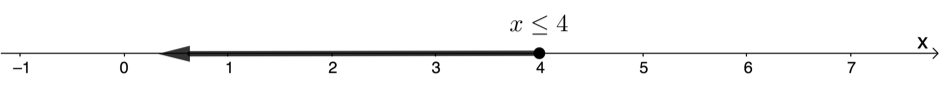Figure 2: Solution to $\scriptsize 3x-4\le 8$

We can see that $\scriptsize x$ does not have a single specific value. $\scriptsize x$ can be any real number from $\scriptsize 4$ or less. We can check this by substituting values into the original inequality to see if the inequality is true.

• $\scriptsize x=4$: $\scriptsize 3(4)-4=12-4=8$ which is less than or equal to $\scriptsize 8$.
• $\scriptsize x=3$: $\scriptsize 3(3)-4=9-4=5$ which is less than or equal to $\scriptsize 8$.
• $\scriptsize x=-4$: $\scriptsize 3(-4)-4=-12-4=-16$ which is less than or equal to $\scriptsize 8$.

But what happens if we try a value for $\scriptsize x$ that is greater than $\scriptsize 4$?

• $\scriptsize x=5$: $\scriptsize 3(5)-4=15-4=11$ which is not less than or equal to $\scriptsize 8$.

### Note### Example 3.1

Solve for $\scriptsize x$ in $\scriptsize 5x-4 \gt 7$ and represent the answer on a number line.

Solution:

In order to solve this inequality, we treat it just like we would an ordinary linear equation.

\scriptsize \begin{align*}5x-4 & \gt 7\\\therefore 5x & \gt 11\quad \text{Add }4\text{ to both sides of the inequality}\\\therefore x & \gt \displaystyle \frac{{11}}{5}\quad \text{Divide both sides of the inequality by }5\end{align*}

We can also check to see if our solution is correct by substituting any value greater than $\scriptsize \displaystyle \frac{{11}}{5}$ (or $\scriptsize 2.2$) into the original inequality to see if it is true. Let’s substitute $\scriptsize x=3$.

LHS: $\scriptsize 5(3)-4=15-4=11$
RHS: $\scriptsize 7$

The LHS is greater than the RHS. Therefore, the inequality is true, and our solution is correct.

In this case $\scriptsize x$ must be greater than but not equal to $\scriptsize \displaystyle \frac{{11}}{5}$. So we use an open dot on the value of $\scriptsize \displaystyle \frac{{11}}{5}$ to indicate that $\scriptsize x$ cannot have this value, and a line to indicate that $\scriptsize x$ can be any real number greater than $\scriptsize \displaystyle \frac{{11}}{2}$.### Interval and set builder notation

Representing the solution to an inequality on a number line is effective but it can take quite a long time to draw. Mathematicians, therefore, use other ways to represent the sets of numbers represented by these solutions.

The first notation is called interval notation. Interval notation uses round and square brackets to indicate if the end point of the range of numbers is included or excluded from the set. Here are some examples to illustrate.

• $\scriptsize (5,11)$: The round brackets indicate that the numbers themselves are not included. This interval includes all real numbers greater than but not equal to $\scriptsize 5$ and less than but not equal to $\scriptsize 11$.
• $\scriptsize [-2,6]$: Square brackets indicate that the numbers are included. This interval includes all real numbers greater than or equal to $\scriptsize -2$ and less than or equal to $\scriptsize 6$.
• $\scriptsize [-7,\infty )$: Round brackets are always used for positive and negative infinity. This interval includes all real numbers greater than or equal to $\scriptsize -7$.

### Note

Interval notation can only be used to represent an interval of real numbers. If the interval or set is only a sub-set of the real numbers, such as integers or natural numbers, we need to use set builder notation.

Set builder notation is slightly more involved than interval notation but it does have the advantage of being able to represent any set of numbers we can think of. The structure of all sets in set builder notation follows the same set of rules. Here is an example:

$\scriptsize \{x|x\in \mathbb{R},\text{ }2 \lt x\le 17\}$

This means the set of all $\scriptsize x$ such that $\scriptsize x$ is a real number ($\scriptsize x$ is an element of the real numbers or $\scriptsize x\in \mathbb{R}\text{ }$) and $\scriptsize x$ is greater than $\scriptsize 2$ and less than or equal to $\scriptsize 17$.

We can represent all the natural numbers between $\scriptsize 4$ and $\scriptsize 20$ (including $\scriptsize 4$ but not including $\scriptsize 20$) in set builder notation like this: .$\scriptsize \{x|x\in \mathbb{N},\text{ }4\le x \lt 20\}$. The set of all $\scriptsize x$ such that $\scriptsize x$ is an element of the natural numbers and $\scriptsize x$ is greater than or equal to $\scriptsize 4$ and less than $\scriptsize 20$.

### Note

Unless otherwise indicated, assume that all sets have $\scriptsize x$ (or whatever number) as an element of the real numbers $\scriptsize \mathbb{R}\text{ }$.### Example 3.2

Solve for $\scriptsize y$ in $\scriptsize 5y+5\ge 3(2y-3)$ and represent the answer on a number line, in interval notation and in set builder notation.

Solution:

\scriptsize \begin{align*}5y+5 & \ge 3(2y-3)\\\therefore 5y+5 & \ge 6y-9\\\therefore -y & \ge -14\end{align*}

We now have the situation where we need to multiply both sides of the inequality by $\scriptsize -1$. When we do so, we need to flip the inequality sign. The reason for this is simple. Consider $\scriptsize 3 \lt 5$. This is true. Now if we multiply (or divide) both sides by $\scriptsize -1$, we get $\scriptsize -3 \lt -5$ which is NOT true. The only way to make the inequality true again, is to flip the inequality sign to get $\scriptsize -3 \gt -5$.

So, in our case, when we multiply (or divide) both sides by $\scriptsize -1$, we need to also flip the inequality sign.

$\scriptsize \ \therefore y \le 14$

Number line:Interval notation: $\scriptsize (-\infty ,14]$. Remember, we cannot include $\scriptsize -\infty$ in the interval so we use a round bracket.

Set builder notation: $\scriptsize \{y|y\in \mathbb{R},\text{ }y\le 14\}$### Exercise 3.1

Solve for $\scriptsize x$ in the following inequalities and represent the solutions on a number line, in interval notation and in set builder notation.

1. $\scriptsize 6x+5 \gt 3x-7$
2. $\scriptsize 5(2x-3)\le -4(x+2)$
3. $\scriptsize \displaystyle \frac{{3x+4}}{4}-\displaystyle \frac{{3x-3}}{3} \ge 1$

The full solutions are at the end of the unit.

## Solve compound inequalities

In the examples we have seen so far, the range of values that the unknown could take to satisfy the original inequality had only one bound; an upper bound or a lower bound.

This means that the possible values for the unknown are either all less than or equal to a certain number (the upper bound) or greater than or equal to a certain number (a lower bound).

But we can also create inequalities that limit the permissible values of the unknown to a specific range between an upper and lower bound. These are called compound inequalities. Here is an example:

$\scriptsize -4 \lt x\le 7$. In this case, $\scriptsize x$ can take any real value between $\scriptsize -4$ (but not including $\scriptsize -4$) and $\scriptsize 7$ (including $\scriptsize 7$). This is represented in interval notation as $\scriptsize (-4,7]$ and on a number line as shown in Figure 4.Figure 4: Number line for $\scriptsize -4 \lt x\le 7$### Example 3.3

Solve for $\scriptsize x$ in the following: $\scriptsize -5\le x+4 \lt 10$ and represent your answer on a number line and using interval notation.

Solution:

We solve compound inequalities the same way as simple inequalities, except that we need to do the same thing to all three parts of the inequality.

\scriptsize \begin{align*}-5 & \le x+4 \lt 10\\\therefore -5-4 & \le x+4-4 \lt 10-4\quad \text{Subtract }4\text{ from all three parts}\\\therefore -9 & \le x \lt 6\end{align*}

We represent this solution using interval notation as $\scriptsize [-9,6)$ and on a number line as follows.### Example 3.4

Solve for $\scriptsize a$ in $\scriptsize 5 \lt 7-a \lt 16$ and represent your answer on a number line and using interval notation.

Solution:

\scriptsize \ \begin{align*}5 & \lt 7-a \lt 16\\\therefore -2 & \lt -a \lt 9\quad \text{Subtract }7\text{ from all three parts}\\\therefore 2 & \gt a \gt -9\quad \text{Divide through by }-1\text{ and change direction of inequalities}\end{align*}

Interval notation: $\scriptsize (-9,2)$

Number line:### Example 3.5

Solve for $\scriptsize x$ in $\scriptsize \ 4x\text{ }+\text{ 7 } \lt \text{ -}3\ \text{or}\ \text{3}x\text{ }+\text{ }3\text{ } \gt \text{ }5$ and represent your answer on a number line and using interval notation.

Solution:

This is a slightly different kind of compound inequality where the two parts are separated. To solve for the unknown, we have to solve each part of the inequality separately and then combine the results.

\scriptsize \ \begin{align*}4x\text{ }+\text{ 7 } \lt \text{ -}3\quad & \text{or}\quad \text{3}x\text{ }+\text{ }3\text{ } \gt \text{ }5\\\therefore 4x \lt -10\quad & \text{or}\quad 3x \gt 2\\\therefore x \lt -\displaystyle \frac{{10}}{4}\quad & \text{or}\quad x \gt \displaystyle \frac{2}{3}\\\therefore x \lt -\displaystyle \frac{5}{2}\quad & \text{or}\quad x \gt \displaystyle \frac{2}{3}\end{align*}

We represent this using interval notation as $\scriptsize (-\infty ,-\displaystyle \frac{5}{2})$ or $\scriptsize (\displaystyle \frac{2}{3},\infty )$.

The number line representation is as follows: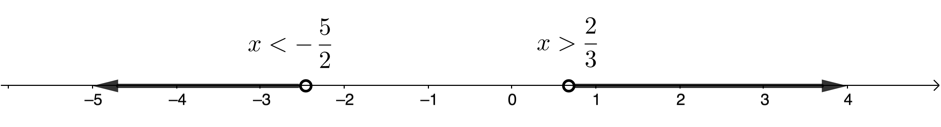### Example 3.6

Solve for $\scriptsize x$ in $\scriptsize -8\le 4-\displaystyle \frac{{2x}}{3}\le 0,\ x\in \mathbb{N}$ and represent your answer on a number line.

Solution:

\scriptsize \ \begin{align*}-8 & \le 4-\displaystyle \frac{{2x}}{3}\le 0\\\therefore -24 & \le 12-2x\le 0\quad \text{Multiply through by }3\\\therefore -36 & \le -2x\le -12\quad \text{Subtract }12\text{ from all three parts}\\\therefore 18 & \ge x\ge 6\quad \text{Divide through by }-2\end{align*}

When we come to represent the solution on a number line, we need to remember that $\scriptsize x\in \mathbb{N}\text{ }$. The number line representation is as follows:In this case, we cannot use a line like we do to represent all the real numbers. We have to use separate closed dots to represent only the natural numbers between and including $\scriptsize 6$ and $\scriptsize 18$.

### Note

Unless told otherwise, you can assume that the unknown can take any real value.### Exercise 3.2

1. Solve for the unknown in each of the following, representing your answers using interval notation and on number lines.
1. $\scriptsize 4 \gt -6x-6 \gt -2$
2. $\scriptsize -5\le \displaystyle \frac{{2a-4}}{3} \lt 1$
3. $\scriptsize \displaystyle \frac{{-3x+6}}{4}\ge 6\quad \text{or}\quad 2x \gt \displaystyle \frac{7}{2}$
4. $\scriptsize 4x+3\le 3\left( {4x+\displaystyle \frac{1}{3}} \right)$
2. Solve for $\scriptsize x$ and represent your answer on a number line and using set builder notation.
.
$\scriptsize \displaystyle \frac{3}{2}(2x-1)\le 2(x+4),\ x\in \mathbb{N}\text{ }$

The full solutions are at the end of the unit.

## Summary

In this unit you have learnt the following:

• How to solve simple linear inequalities.
• How to solve compound inequalities.
• How to represent the solution to an inequality on a number line.
• How to represent the solution to an inequality using interval notation.

# Unit 3: Assessment

#### Suggested time to complete: 30 minutes

1. Solve for $\scriptsize x$ in the following inequalities, representing your answer on number lines.
1. $\scriptsize -2\le x+6 \lt 9$
2. $\scriptsize 2-3x\le 3-5x$
3. $\scriptsize -5 \lt 1+3x\le 10,\ x\in \mathbb{Z}\text{ }$
2. Solve for the unknown in each case, representing your answers using interval notation and set builder notation.
1. $\scriptsize \displaystyle \frac{{4y-2}}{6} \gt 2y+1$
2. $\scriptsize \displaystyle \frac{{1-s}}{3}+\displaystyle \frac{{1-s}}{2}\le 5$
3. $\scriptsize \ -\displaystyle \frac{{2x+6}}{3} \gt 4\quad \text{or}\quad \displaystyle \frac{{3x-3}}{4} \gt 0$
4. $\scriptsize \displaystyle \frac{{7x+3}}{4} \lt 4(2x-7)$

The full solutions are at the end of the unit.

# Unit 3: Solutions

### Exercise 3.1

1. .
\scriptsize \begin{align*}6x+5 & \gt 3x-7\\\therefore 3x & \gt -12\\\therefore x & \gt -4\end{align*}
.
Number line: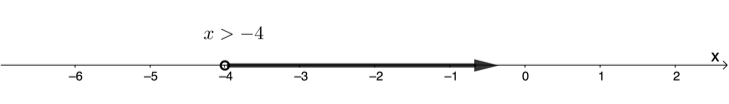Interval notation: $\scriptsize (-4,\infty )$
Set builder notation: $\scriptsize \{x|x\in \mathbb{R},\text{ }x \gt -4\}$
2. .
\scriptsize \begin{align*}5(2x-3) & \le -4(x+2)\\\therefore 10x-15 & \le -4x-8\\\therefore 14x & \le 7\\\therefore x & \le \displaystyle \frac{1}{2}\end{align*}
.
Number line: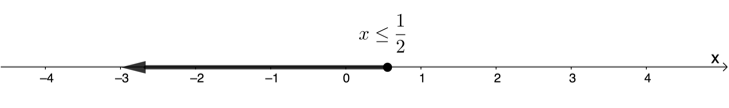Interval notation: $\scriptsize (-\infty ,\displaystyle \frac{1}{2}]$
Set builder notation: $\scriptsize \{x|x\in \mathbb{R},\text{ }x\le \displaystyle \frac{1}{2}\}$
3. .
\scriptsize \begin{align*}\displaystyle \frac{{3x+4}}{4}-\displaystyle \frac{{3x-3}}{3} & \ge 1\\\therefore 3(3x+4)-4(3x-3) & \ge 12\\\therefore 9x+12-12x+12 & \ge 12\\\therefore -3x & \ge -12\\\therefore x & \le 4\end{align*}
.
Number line: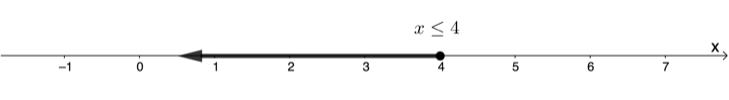Interval notation: $\scriptsize (-\infty ,4]$
Set builder notation: $\scriptsize \{x|x\in \mathbb{R},\text{ }x\le 4\}$

Back to Exercise 3.1

### Exercise 3.2

1. .
1. .
\scriptsize \begin{align*}4 & \gt -6x-6 \gt -2\\\therefore 10 & \gt -6x \gt 4\\\therefore -\displaystyle \frac{{10}}{6} & \lt x \lt -\displaystyle \frac{4}{6}\\\therefore -\displaystyle \frac{5}{3} & \lt x \lt -\displaystyle \frac{2}{3}\end{align*}
.
Interval notation: $\scriptsize (-\displaystyle \frac{5}{3},-\displaystyle \frac{2}{3})$
Number line:2. .
\scriptsize \begin{align*}-5 & \le \displaystyle \frac{{2a-4}}{3} \lt 1\\\therefore -15 & \le 2a-4 \lt 3\\\therefore -11 & \le 2a \lt 7\\\therefore -\displaystyle \frac{{11}}{2} & \le a \lt \displaystyle \frac{7}{2}\end{align*}
.
Interval notation: $\scriptsize [-\displaystyle \frac{{11}}{2},\displaystyle \frac{7}{2})$
Number line:3. .
\scriptsize \begin{align*}\displaystyle \frac{{-3x+6}}{4}\ge 6\quad & \text{or}\quad 2x \gt \displaystyle \frac{7}{2}\\\therefore -3x+6\ge 24\quad & \text{or}\quad x \gt \displaystyle \frac{7}{4}\\\therefore -3x\ge 18\quad & \text{or}\quad x \gt \displaystyle \frac{7}{4}\\\therefore x\le -6\quad & \text{or}\quad x \gt \displaystyle \frac{7}{4}\end{align*}
.
Interval notation: $\scriptsize (-\infty ,-6]$ or $\scriptsize (\displaystyle \frac{7}{4},\infty )$
Number line:4. .
\scriptsize \begin{align*}4x+3 & \le 3\left( {4x+\displaystyle \frac{1}{3}} \right)\\\therefore 4x+3 & \le 12x+1\quad \text{Subtract }12x\text{ from both sides of the inequality}\\\therefore -8x & \le -2\\\therefore x & \ge \displaystyle \frac{1}{4}\end{align*}
.
Interval notation: $\scriptsize [\displaystyle \frac{1}{4},\infty )$
Number line: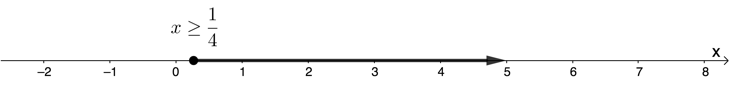2. .
\scriptsize \begin{align*}\displaystyle \frac{3}{2}(2x-1) & \le 2(x+4),\ x\in \mathbb{N}\text{ }\\\therefore 3(2x-1) & \le 4(x+4),\ x\in \mathbb{N}\text{ }\\\therefore 6x-3 & \le 4x+16,\ x\in \mathbb{N}\text{ }\\\therefore 2x & \le 19,\ x\in \mathbb{N}\text{ }\\\therefore x & \le \displaystyle \frac{{19}}{2},\ x\in \mathbb{N}\text{ }\end{align*}
.
$\scriptsize x$ can only be a natural number. Therefore, the first permitted solution less than $\scriptsize \displaystyle \frac{{19}}{2}$ (or $\scriptsize 9.5$) is $\scriptsize 9$. Even though there is no specific lower bound, a lower bound of $\scriptsize 1$ is enforced by the fact that $\scriptsize x\in \mathbb{N}\text{ }$.
Number line: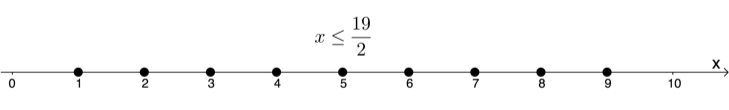Set builder notation: Set builder notation: $\scriptsize \{x|x\in \mathbb{N},x\le \displaystyle \frac{{19}}{2}\}$

Back to Exercise 3.2

### Unit 3: Assessment

1. .
1. .
\scriptsize \begin{align*}-2\le x+6 \lt 9\\\therefore -8 & \le x \lt 3\end{align*}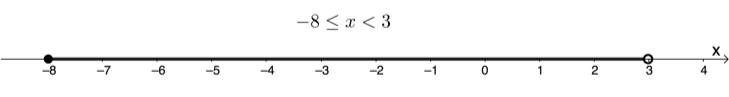2. .
\scriptsize \begin{align*}2-3x\le 3-5x\\\therefore -3x & \le 1-5x\quad \text{Subtract }2\text{ from both sides of the inequality}\\\therefore 2x & \le 1\quad \text{Add }5x\text{ to both sides of the inequality}\\\therefore x & \le \displaystyle \frac{1}{2}\end{align*}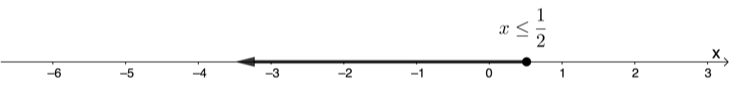3. .
\scriptsize \begin{align*}-5 & \lt 1+3x\le 10,x\in \mathbb{Z}\text{ }\\\therefore -6 & \lt 3x\le 9,x\in \mathbb{Z}\text{ }\\\therefore -2 & \lt x\le 3,x\in \mathbb{Z}\text{ }\end{align*}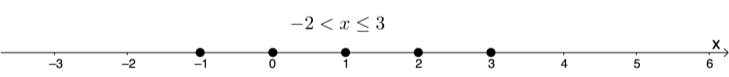2. .
1. .
\scriptsize \begin{align*}\displaystyle \frac{{4y-2}}{6} & \gt 2y+1\\\therefore 4y-2 & \gt 12y+6\\\therefore -8y & \gt 8\\\therefore y & \lt -1\end{align*}
Interval notation: $\scriptsize (-\infty ,-1)$
Set builder notation: $\scriptsize \{y|y\in \mathbb{R},y \lt -1\}$
2. .
\scriptsize \begin{align*}\displaystyle \frac{{1-s}}{3}+\displaystyle \frac{{1-s}}{2} & \le 5\\\therefore 2(1-s)+3(1-s) & \le 30\\\therefore 2-2s+3-3s & \le 30\\\therefore -5s & \le 25\\\therefore s & \ge -5\end{align*}
Interval notation: $\scriptsize [-5,\infty )$
Set builder notation: $\scriptsize \{s|s\in \mathbb{R},s\ge -5\}$
3. .
\scriptsize \begin{align*}-\displaystyle \frac{{2x+6}}{3} \gt 4\quad & \text{or}\quad \displaystyle \frac{{3x-3}}{4} \gt 0\\\therefore -(2x+6) \gt 12\quad & \text{or}\quad 3x-3 \gt 0\\\therefore -2x-6 \gt 12\quad & \text{or}\quad 3x-3\\\therefore -2x \gt 18\quad & \text{or}\quad x \gt 1\\\therefore x-9\quad & \text{or}\quad x \gt 1\end{align*} Interval notation: $\scriptsize (-\infty ,-9)$ or $\scriptsize (1,\infty )$ Set builder notation: $\scriptsize \{x|x\in \mathbb{R},x \lt -9,x \gt 1\}$</li>
4. .
\scriptsize \begin{align*}\displaystyle \frac{{7x+3}}{4} & 4(2x-7)\\\therefore 7x+3 & 16(2x-7)\\\therefore 7x+3 & 32x-112\\\therefore -25x & -115\\\therefore x & \gt \displaystyle \frac{{115}}{{25}}\\\therefore x & \gt \displaystyle \frac{{23}}{5}\end{align*} Interval notation: $\scriptsize (\displaystyle \frac{{23}}{5},\infty )$ Set builder notation: $\scriptsize \{x|x\in \mathbb{R},x \gt \displaystyle \frac{{23}}{5}\}$</li>

Back to Unit 3: Assessment